Courses

# Theories of Column & Theories of Failure - MCQ Test 2

## 25 Questions MCQ Test RRB JE for Mechanical Engineering | Theories of Column & Theories of Failure - MCQ Test 2

Description
This mock test of Theories of Column & Theories of Failure - MCQ Test 2 for Mechanical Engineering helps you for every Mechanical Engineering entrance exam. This contains 25 Multiple Choice Questions for Mechanical Engineering Theories of Column & Theories of Failure - MCQ Test 2 (mcq) to study with solutions a complete question bank. The solved questions answers in this Theories of Column & Theories of Failure - MCQ Test 2 quiz give you a good mix of easy questions and tough questions. Mechanical Engineering students definitely take this Theories of Column & Theories of Failure - MCQ Test 2 exercise for a better result in the exam. You can find other Theories of Column & Theories of Failure - MCQ Test 2 extra questions, long questions & short questions for Mechanical Engineering on EduRev as well by searching above.
QUESTION: 1

### Match 4 correct pairs between list I and List II for the questions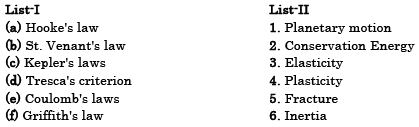[GATE-1994]

Solution:

St. Venant's law: Maximum principal strain theory

QUESTION: 2

### Which theory of failure will you use for aluminium components under steady loading? [GATE-1999]

Solution:

Aluminium is a ductile material so use maximum shear stress theory

QUESTION: 3

### According to Von-Mises' distortion energy theory, the distortion energy under three dimensional stress state is represented by

Solution: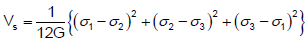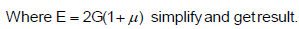QUESTION: 4

A small element at the critical section of a component is in a bi-axial state of stress with the two principal stresses being 360 MPa and 140 MPa. The maximum working stress according to Distortion Energy Theory is:

[GATE-1997]

Solution:

According to distortion energy theory if maximum stress (σt) then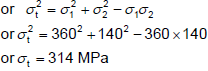QUESTION: 5

Match List-I (Theory of Failure) with List-II (Predicted Ratio of Shear Stress to Direct Stress at Yield Condition for Steel Specimen) and select the correct answer using the code given below the Lists: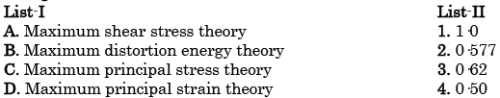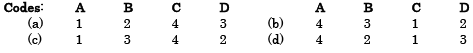[IES-2006]

Solution:
QUESTION: 6

A circular solid shaft is subjected to a bending moment of 400 kNm and a twisting moment of 300 kNm. On the basis of the maximum principal stress theory, the direct stress is σ and according to the maximum shear stress theory, the shear stress is τ . The ratio σ/τ is:

[IES-2000]

Solution: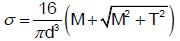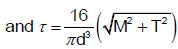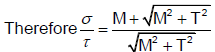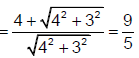QUESTION: 7

Design of shafts made of brittle materials is based on

[IES-1993]

Solution:

Rankine's theory or maximum principle stress theory is most commonly used for
brittle materials.

QUESTION: 8

According to the maximum shear stress theory of failure, permissible twisting moment in a circular shaft is 'T'. The permissible twisting moment will the same shaft as per the maximum principal stress theory of failure will be:

[IES-1998: ISRO-2008]

Solution:principalstressesfor only thisshear stressare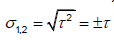maximum principal stress theory of failuregives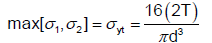QUESTION: 9

A cold roller steel shaft is designed on the basis of maximum shear stress theory. The principal stresses induced at its critical section are 60 MPa and - 60 MPa respectively. If the yield stress for the shaft material is 360 MPa, the factor of safety of the design is

[IES-2002]

Solution:
QUESTION: 10

For a two-dimensional state stress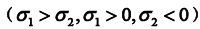the designed values are most conservative if which one of the following failure theories were used?

[IES-1998]

Solution: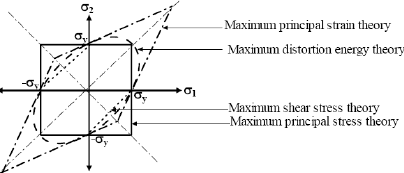Graphical comparison of different failure theories
Above diagram shows that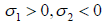will occur at 4th quadrant and most
conservative design will be maximum shear stress theory.

QUESTION: 11

Who postulated the maximum distortion energy theory?

[IES-2008]

Solution:

Maximum shear stress theory → Tresca
Maximum principal stress theory → Rankine
Maximum principal strain theory → St. Venant
Maximum shear strain energy theory → Mises – Henky

QUESTION: 12

If σy is the yield strength of a particular material, then the distortion energy theory is expressed as

[IES-1994]

Solution:
QUESTION: 13

A transmission shaft subjected to bending loads must be designed on the basis of

[IES-1996]

Solution:
QUESTION: 14

A rod having cross-sectional area 100 x 10- 6 m2 is subjected to a tensile load. Based on the Tresca failure criterion, if the uniaxial yield stress of the material is 200 MPa, the failure load is:

[IES-2001]

Solution:

Tresca failure criterion is maximum shear stress theory.

Weknowthat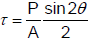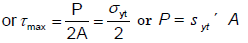QUESTION: 15

Who postulated the maximum distortion energy theory?

[IES-2008]

Solution:
QUESTION: 16

For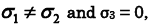what is the physical boundary for Rankine failure theory?

[IAS-2004]

Solution:

Rankine failure theory or
Maximum principle stress theory.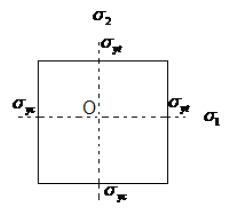QUESTION: 17

Which one of the following graphs represents Mises yield criterion?

IAS-1996]

Solution:
QUESTION: 18

The maximum distortion energy theory of failure is suitable to predict the failure of which one of the following types of materials?

[IES-2004]

Solution:
QUESTION: 19

The ratio of Euler's buckling loads of columns with the same parameters having (i) both ends fixed, and (ii) both ends hinged is:

[GATE-1998; 2002; IES-2001]

Solution:

Euler‟s buckling loads of columns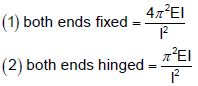QUESTION: 20

For a long slender column of uniform cross section, the ratio of critical buckling load for the case with both ends clamped to the case with both ends hinged is

[GATE-2012]

Solution:
QUESTION: 21

Four columns of same material and same length are of rectangular cross-section of same breadth b. The depth of the cross-section and the end conditions are, however different are given as follows: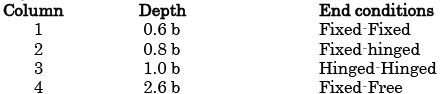Which of the above columns Euler buckling load maximum?

[IES-2004]

Solution:
QUESTION: 22

Assertion (A): A long column of square cross-section has greater buckling stability than that of a column of circular cross-section of same length, same material, same end conditions and same area of cross-section.

Reason (R): The second moment of area of a column of circular cross-section is smaller than that of a column of square cross section having the same area.

[IAS-1998]

Solution:
QUESTION: 23

For which one of the following columns, Euler buckling load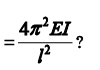[IAS-1999; 2004]

Solution:
QUESTION: 24

What is the expression for the crippling load for a column of length „l‟ with one end fixed and other end free?

[IES-2006; GATE-1994]

Solution:
QUESTION: 25

Euler's formula gives 5 to 10% error in crippling load as compared to experimental results in practice because:

[IES-1998]

Solution: